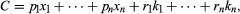Theory of production | economics | Britannica

# Theory of production

economics
Alternative Title: production theory

Theory of production, in economics, an effort to explain the principles by which a business firm decides how much of each commodity that it sells (its “outputs” or “products”) it will produce, and how much of each kind of labour, raw material, fixed capital good, etc., that it employs (its “inputs” or “factors of production”) it will use. The theory involves some of the most fundamental principles of economics. These include the relationship between the prices of commodities and the prices (or wages or rents) of the productive factors used to produce them and also the relationships between the prices of commodities and productive factors, on the one hand, and the quantities of these commodities and productive factors that are produced or used, on the other.

The various decisions a business enterprise makes about its productive activities can be classified into three layers of increasing complexity. The first layer includes decisions about methods of producing a given quantity of the output in a plant of given size and equipment. It involves the problem of what is called short-run cost minimization. The second layer, including the determination of the most profitable quantities of products to produce in any given plant, deals with what is called short-run profit maximization. The third layer, concerning the determination of the most profitable size and equipment of plant, relates to what is called long-run profit maximization.

## The production function

However much of a commodity a business firm produces, it endeavours to produce it as cheaply as possible. Taking the quality of the product and the prices of the productive factors as given, which is the usual situation, the firm’s task is to determine the cheapest combination of factors of production that can produce the desired output. This task is best understood in terms of what is called the production function, i.e., an equation that expresses the relationship between the quantities of factors employed and the amount of product obtained. It states the amount of product that can be obtained from each and every combination of factors. This relationship can be written mathematically as y = f (x1, x2, . . ., xn; k1, k2, . . ., km). Here, y denotes the quantity of output. The firm is presumed to use n variable factors of production; that is, factors like hourly paid production workers and raw materials, the quantities of which can be increased or decreased. In the formula the quantity of the first variable factor is denoted by x1 and so on. The firm is also presumed to use m fixed factors, or factors like fixed machinery, salaried staff, etc., the quantities of which cannot be varied readily or habitually. The available quantity of the first fixed factor is indicated in the formal by k1 and so on. The entire formula expresses the amount of output that results when specified quantities of factors are employed. It must be noted that though the quantities of the factors determine the quantity of output, the reverse is not true, and as a general rule there will be many combinations of productive factors that could be used to produce the same output. Finding the cheapest of these is the problem of cost minimization.

The cost of production is simply the sum of the costs of all of the various factors. It can be written:in which p1 denotes the price of a unit of the first variable factor, r1 denotes the annual cost of owning and maintaining the first fixed factor, and so on. Here again one group of terms, the first, covers variable cost (roughly“direct costs” in accounting terminology), which can be changed readily; another group, the second, covers fixed cost (accountants’ “overhead costs”), which includes items not easily varied. The discussion will deal first with variable cost.

The principles involved in selecting the cheapest combination of variable factors can be seen in terms of a simple example. If a firm manufactures gold necklace chains in such a way that there are only two variable factors, labour (specifically, goldsmith-hours) and gold wire, the production function for such a firm will be y = f (x1, x2; k), in which the symbol k is included simply as a reminder that the number of chains producible by x1 feet of gold wire and x2 goldsmith-hours depends on the amount of machinery and other fixed capital available. Since there are only two variable factors, this production function can be portrayed graphically in a figure known as an isoquant diagram (Figure 1). In the graph, goldsmith-hours per month are plotted horizontally and the number of feet of gold wire used per month vertically. Each of the curved lines, called an isoquant, will then represent a certain number of necklace chains produced. The data displayed show that 100 goldsmith-hours plus 900 feet of gold wire can produce 200 necklace chains. But there are other combinations of variable inputs that could also produce 200 necklace chains per month. If the goldsmiths work more carefully and slowly, they can produce 200 chains from 850 feet of wire; but to produce so many chains more goldsmith-hours will be required, perhaps 130. The isoquant labelled “200” shows all the combinations of the variable inputs that will just suffice to produce 200 chains. The other two isoquants shown are interpreted similarly. It is obvious that many more isoquants, in principle an infinite number, could also be drawn. This diagram is a graphic display of the relationships expressed in the production function.Figure 1: Isoquant diagram of hours of labour and feet of gold wire used per month.Encyclopædia Britannica, Inc.
Theory of production